MTH4140 Home

# Planar Graphs and Euler's Formula

## Planar graphs

A graph is planar if it can be drawn in such a way that edges do not cross.

Definition A graph is planar if it is isomorphic to a graph whose vertices are points in a plane and whose edges can be represented by simple curves with non-intersecting interiors.

Problem 1

Prove that the following three graphs are planar.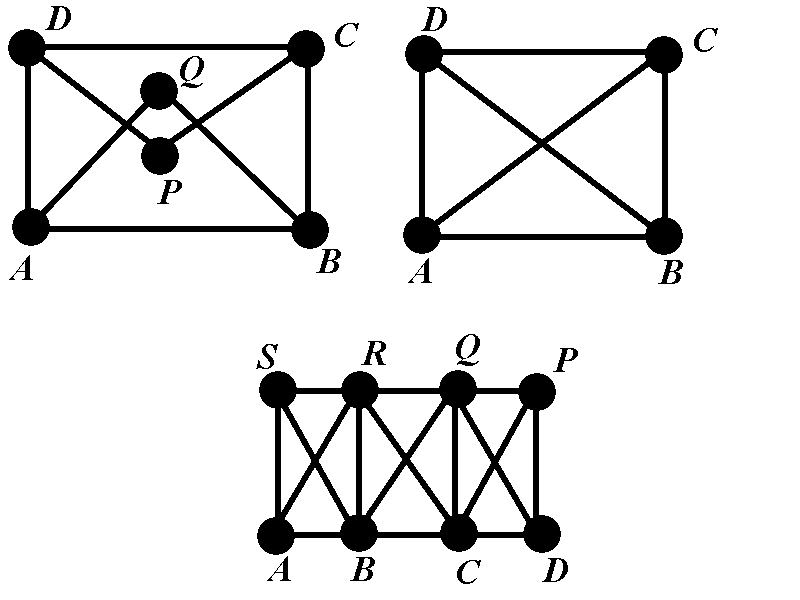The complete graph $$K_5$$ with $$5$$ vertices is not planar. You can try as much as you want but you can‘t manage to draw that graph without intersecting edges. Similarly, the complete bipartite graph $$K_{3,3}$$ is not planar. We will later use Euler's theorem to prove that these two graphs are not planar.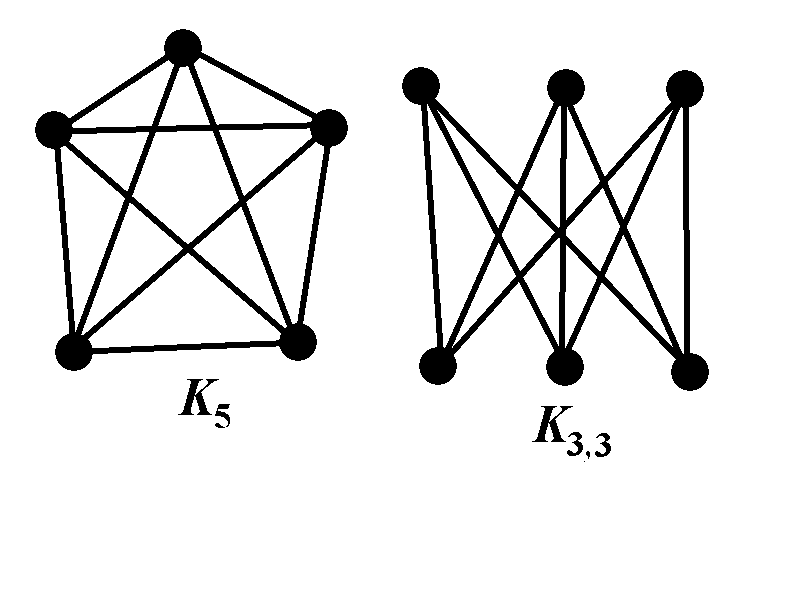## Faces of planar graphs

A face of a planar graph is a connected region in the plane bounded by the edges of the graph.

Problem 2

How many faces does the following graph have?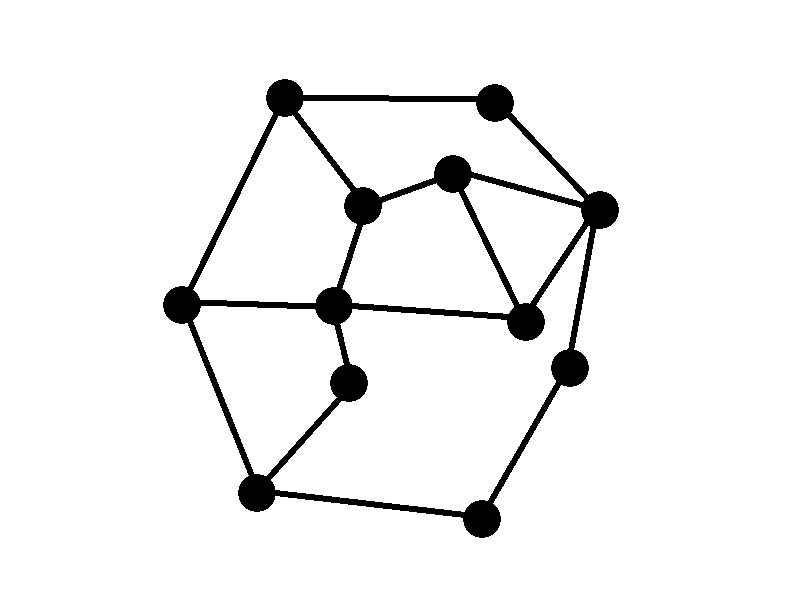## Triangulation of planar graphs

A triangulation of a planar graph consists of adding edges to the graph until every face is a triangle.

Problem 3

Determine a triangulation of the graph from problem 2.

## Euler's formula

Theorem (Euler$${}^{\prime}$$s formula) If a connected planar graph has $$n$$ vertices, $$e$$ edges, and $$f$$ faces, then $n+f=e+2.$

The proof is on page 241 of the textbook.

We can now prove that $$K_5$$ cannot be planar. The number of vertices of $$K_5$$ is $$n=5$$. The number of edges is $$e=10$$. Let us assume that $$K_5$$ is planar. Then it must have faces. Let us denote by $$f$$ the number of faces. Let us denote by $$e_1$$, $$e_2$$, $$\dots$$, $$e_f$$ the number of edges on the faces. Observe that $e_1+e_2+\cdots+e_f=2e.$ The last equality is proved in similar way as the handshake theorem: when we add the edges for all the faces and create the sum $$e_1+e_2+\cdots+e_f$$ then each edge is counted exactly twice because each edge belongs to exactly two faces. Since each face has at least three edges we have $$e_1+e_2+\cdots+e_f\geq 3f$$. Therefore $$2e\geq 3f$$. From Euler's formula we obtain $e+2=n+f\leq n+\frac23e.$ The inequality $$n+\frac23e\geq e+2$$ is equivalent to $$n\geq \frac e3+2$$ which is the same as $$3n\geq e+6$$. This is not possible because for $$n=5$$ and $$e=10$$ we have $$3n=15$$ while $$e+6=16$$ and $$15\geq 16$$ is just wrong. This is a contradiction.

The proof that $$K_{3,3}$$ is not planar is similar. If a graph is triangle-free then the inequality $$3n\geq e+6$$ can be improved to $$2n\geq e+4$$. Since the graph $$K_{3,3}$$ is bipartite, and hence triangle-free, the $$2n\geq e+4$$. However, for $$K_{3,3}$$ we have $$n=6$$ and $$e=9$$. The number $$2n$$ is equal to $$12$$, while $$e+4$$ is equal to $$13$$ and $$12\geq 13$$ is a contradiction.

## Polyhedron

A polyhedron is a solid object in 3 dimensions. Every convex polyhedron corresponds to a planar graph. We can see that in the following way: First we blow the air in the polyhedron until it becomes a sphere.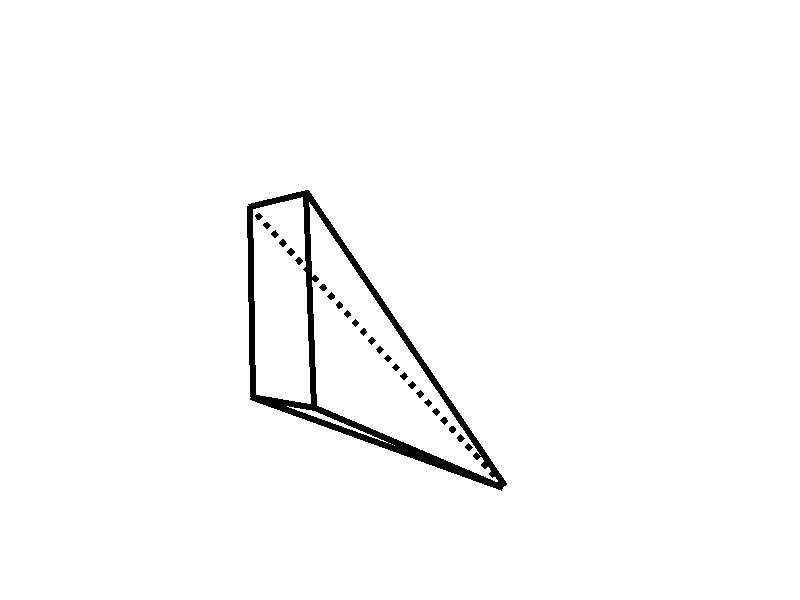$$\;$$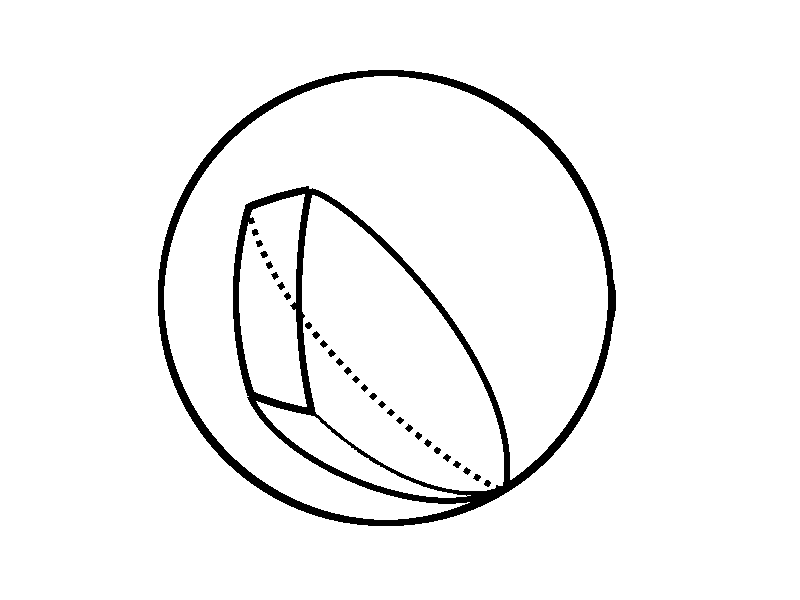Then we find a point $$P$$ on the sphere that is not an edge or a vertex of the polyhedron. We then rotate our point of view so that $$P$$ is the top of the sphere, or north pole for those of you familiar with geography. We then find one plane far away below the south pole. Then we find intersections of this plane with all lines connecting $$P$$ with points of the polyhedron. The obtained projection is a planar graph.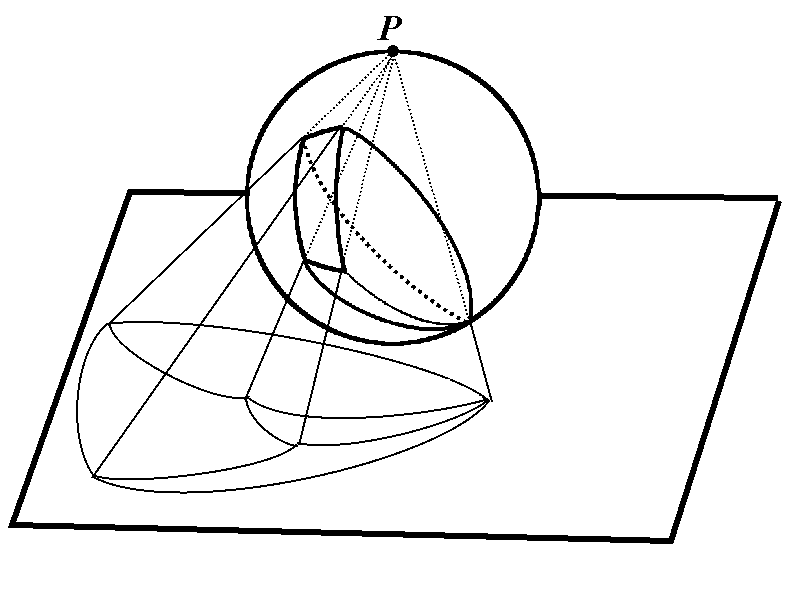$$\;$$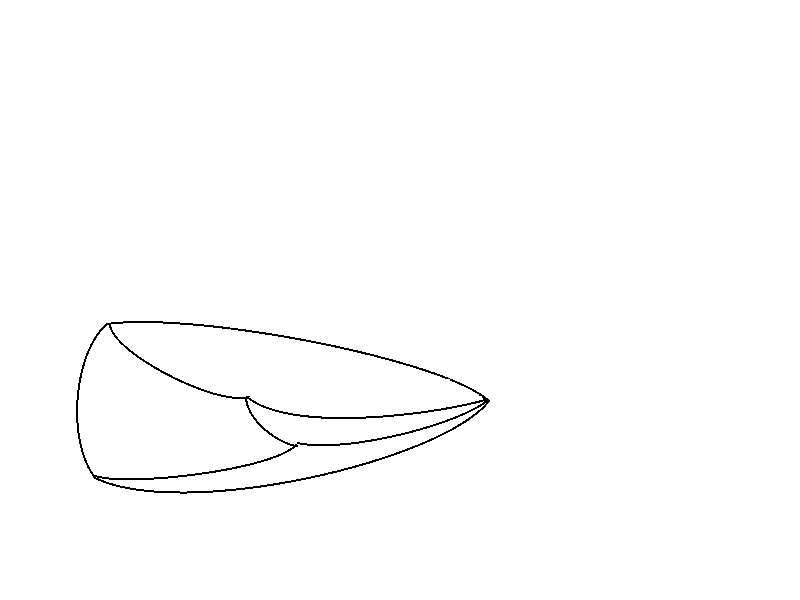Problem 4

Prove that at least one face of a convex polyhedron has fewer than or equal to $$5$$ edges.